# Generalized Forward-Backward Proximal Splitting¶

Important: Please read the installation page for details about how to install the toolboxes. $\newcommand{\dotp}{\langle #1, #2 \rangle}$ $\newcommand{\enscond}{\lbrace #1, #2 \rbrace}$ $\newcommand{\pd}{ \frac{ \partial #1}{\partial #2} }$ $\newcommand{\umin}{\underset{#1}{\min}\;}$ $\newcommand{\umax}{\underset{#1}{\max}\;}$ $\newcommand{\umin}{\underset{#1}{\min}\;}$ $\newcommand{\uargmin}{\underset{#1}{argmin}\;}$ $\newcommand{\norm}{\|#1\|}$ $\newcommand{\abs}{\left|#1\right|}$ $\newcommand{\choice}{ \left\{ \begin{array}{l} #1 \end{array} \right. }$ $\newcommand{\pa}{\left(#1\right)}$ $\newcommand{\diag}{{diag}\left( #1 \right)}$ $\newcommand{\qandq}{\quad\text{and}\quad}$ $\newcommand{\qwhereq}{\quad\text{where}\quad}$ $\newcommand{\qifq}{ \quad \text{if} \quad }$ $\newcommand{\qarrq}{ \quad \Longrightarrow \quad }$ $\newcommand{\ZZ}{\mathbb{Z}}$ $\newcommand{\CC}{\mathbb{C}}$ $\newcommand{\RR}{\mathbb{R}}$ $\newcommand{\EE}{\mathbb{E}}$ $\newcommand{\Zz}{\mathcal{Z}}$ $\newcommand{\Ww}{\mathcal{W}}$ $\newcommand{\Vv}{\mathcal{V}}$ $\newcommand{\Nn}{\mathcal{N}}$ $\newcommand{\NN}{\mathcal{N}}$ $\newcommand{\Hh}{\mathcal{H}}$ $\newcommand{\Bb}{\mathcal{B}}$ $\newcommand{\Ee}{\mathcal{E}}$ $\newcommand{\Cc}{\mathcal{C}}$ $\newcommand{\Gg}{\mathcal{G}}$ $\newcommand{\Ss}{\mathcal{S}}$ $\newcommand{\Pp}{\mathcal{P}}$ $\newcommand{\Ff}{\mathcal{F}}$ $\newcommand{\Xx}{\mathcal{X}}$ $\newcommand{\Mm}{\mathcal{M}}$ $\newcommand{\Ii}{\mathcal{I}}$ $\newcommand{\Dd}{\mathcal{D}}$ $\newcommand{\Ll}{\mathcal{L}}$ $\newcommand{\Tt}{\mathcal{T}}$ $\newcommand{\si}{\sigma}$ $\newcommand{\al}{\alpha}$ $\newcommand{\la}{\lambda}$ $\newcommand{\ga}{\gamma}$ $\newcommand{\Ga}{\Gamma}$ $\newcommand{\La}{\Lambda}$ $\newcommand{\si}{\sigma}$ $\newcommand{\Si}{\Sigma}$ $\newcommand{\be}{\beta}$ $\newcommand{\de}{\delta}$ $\newcommand{\De}{\Delta}$ $\newcommand{\phi}{\varphi}$ $\newcommand{\th}{\theta}$ $\newcommand{\om}{\omega}$ $\newcommand{\Om}{\Omega}$

This tour explores the use of an advanced non-smooth optimization scheme to handle composite inverse problems resolution.

This tour is written by <http://www.ceremade.dauphine.fr/~raguet/ Hugo Raguet>.

We use a proximal splitting algorithm detailed in

Hugo Raguet, Jalal M. Fadili and Gabriel Peyre, Generalized Forward-Backward Splitting Algorithm, <http://arxiv.org/abs/1108.4404 preprint arXiv:1108.4404v2>, 2011.

In :
addpath('toolbox_signal')


## Convex Optimization with Generalized Forward-Backward Splitting¶

We consider general optimization problems of the form $$\umin{x} F(x) + \sum_{i=1}^n G_i(x)$$ where $F$ is a convex, differentiable function, with Lipschitz-continuous gradient and the $G_i$'s are convex functions

To use proximal algorithm, one should be able to compute the proximity operator of the $G_i$'s, defined as: $$\text{prox}_{\gamma G_i}(x) = \uargmin{y} \frac{1}{2} \norm{x-y}^2 + \gamma G_i(y).$$

The algorithm reads: $$\text{for all } i, \quad z_{i,t+1} = z_{i,t} + \text{prox}_{n \gamma G_i}( 2 x_t - z_{i,t} - \gamma \nabla F(x_t) ) - x_t$$ $$x_{t+1} = \frac{1}{n} \sum_{i=1}^n z_{i,t+1}.$$

It can be shown that if $0 < \gamma < 2 \beta$ where $\frac{1}{\beta}$ is a Lipschitz constant of $\nabla F$, then ${(x_t)}_t$ converges to a minimizer of $F + \sum_{i=1}^n G_i$.

## Joint Inpainting and Deblurring¶

We consider a linear imaging operator $\Phi : f \mapsto \Phi(f)$ that maps high resolution images to low dimensional observations. Here we consider a composition of a pixel masking operator $M$ and of a blurring operator $K$.

Load an image $f_0$.

In :
name = 'lena';
N = 256;
f0 = rescale(crop(f0,N));


Display it.

In :
clf
imageplot(f0);First, we define the masking operator. It is a projector on the set of valid pixels. It is equivalently implemented as a diagonal operator that multiplies the image by a binary mask

In :
rho_M = .7;


Then, we define the blurring operator $K$, which is is a convolution with a kernel $k$: $K(f) = k \star f$.

We load a gaussian kernel $k$ of variance $\si_K=2$. Note that the Young's inequality gives for any $f$ $$\norm{f \star k}_2 \leq \norm{f}_2 \norm{k}_1$$ We normalize $k$ so that $\norm{k}_1=1$ which ensures $\norm{K}=1$.

In :
sig_K = 2;
[X,Y] = meshgrid( [0:N/2-1 -N/2:-1] );
k = exp( - (X.^2+Y.^2) / (2*sig_K^2) );
k = k./sum(abs(k(:)));


A convolution is equivalent to a multiplication in the Fourier domain. $$\Ff(f \star k) = \Ff(f) \cdot \Ff(k), \quad\text{so that}\quad K(f) = \Ff^{-1}(\Ff(f) \cdot \Ff(k))$$ where $\Ff$ is the 2-D Fourier transform.

We thus implement $K$ using the Fourier transform of the kernel.

In :
Fk = fft2(k);
K = @(f) real( ifft2( fft2(f).*Fk ) );


The masking and blurring operator $\Phi = M \circ K$.

In :
Phi = @(f)M(K(f));


Compute the observations $y = \Phi f_0 + w$, where $w$ is a Gaussian white noise of variance $\si_w$.

In :
sig_w = .025;
y = Phi(f0) + sig_w*randn(N,N);


Display it.

In :
clf
imageplot(y);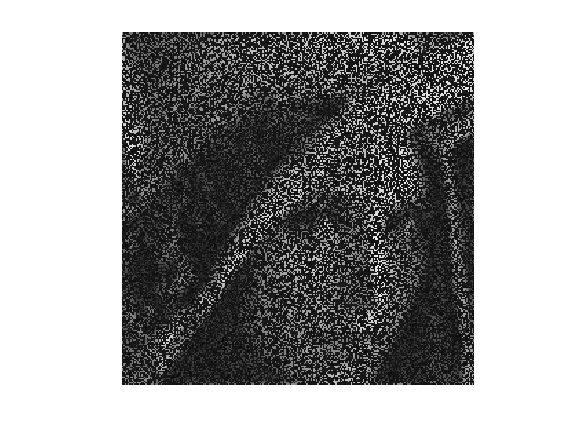## Splitting Total Variation Regularization¶

We want to solve the noisy inverse problem $y = \Phi x + w$ using a total variation regularization: $$\umin{x} \frac{1}{2} \norm{y - \Phi x}^2 + \la \norm{x}_{\text{TV}}.$$

The total variation pseudo-norm is defined as the sum over all pixels $p=(p_1,p_2)$ of the norm of the image gradient $\text{grad}(x)_p$ at $p$.

The gradient is computed using finite differences $$\text{grad} \, : \, \choice{ \mathbb{R}^{N \times N} \rightarrow \mathbb{R}^{N \times N \times 2} \\ x \mapsto ( x \star h_1, x \star h_2 ), }$$ where $h_1$ and $h_2$ are two 2-D filters.

One usually computes the gradient using finite differences along vertical and horizontal directions. This corresponds to convolutions $\text{grad}(x) = (x \star h_1,x \star h_2) \in \RR^{N \times N \times 2}$ where $$h_1 = \begin{pmatrix} 0 & 0 & 0 \\ 0 & -1 & 0 \\ 0 & 1 & 0 \end{pmatrix} \qandq h_2 = \begin{pmatrix} 0 & 0 & 0 \\ 0 & -1 & 1 \\ 0 & 0 & 0 \end{pmatrix}.$$ Note that we write 2-D filters as $3 \times 3$ matrices, which implicitely assumes that the central index (position 0) is at the center of this matrix.

Following the method introduced in:

P. L. Combettes and J.-C. Pesquet, A proximal decomposition method for solving convex variational inverse problems, Inverse Problems, vol. 24, no. 6, article ID 065014, 27 pp., December 2008.

we use diagonal filters: $$h_1 = \begin{pmatrix} 0 & 0 & 0 \\ 0 & -1 & 0 \\ 0 & 0 & 1 \end{pmatrix} \qandq h_2 = \begin{pmatrix} 0 & 0 & 0 \\ 0 & 0 & 1 \\ 0 &-1 & 0 \end{pmatrix}$$ is more interesting for us, since those kernels are orthogonals: they do not overlap. We will use these diagonal filters to split the total variation into simpler functions.

The TV norm can be written as the $\ell_1-\ell_2$ norm $G(u)$ of the gradient $u=\text{grad}(x)$ $$\norm{x}_{\text{TV}} = G( \text{grad}(x) ) \qwhereq G(u) = \sum_{p} \norm{u_p} = \sum_p \sqrt{ {u_{1,p}}^2 + {u_{2,p}}^2 }$$ where $u = ( (u_{1,p},u_{2,p}) )_{p} \in \RR^{N \times N \times 2}$ is a vector field.

The proximal operator of $G$ is the soft thresholding of the norm of each vector $u_p \in \RR^2$ in the vector field, which corresponds to $$\text{prox}_{\gamma G}(u)_{p} = \max\pa{0, 1-\frac{\gamma}{\norm{u_{p}}}} u_{p} .$$ If the norm of $u_{p}$ is lower than $\gamma$, then $u_p$ is set to zero; otherwise, both coordinates of $u_p$ are shrinked by the same factor.

We define the proximity operator of $G$.

In :
G = @(u) sqrt( sum( u.^2, 3 ) );
proxG = @(u,gamma) repmat( max(0,1 - gamma./G(u) ), [1 1 2] ).*u;


We would like to compute the proximity operator of $\norm{\cdot}_{\text{TV}} = G \circ \text{grad}$. This is much more complicated than computing the proximity operator of $G$ because the linear operator $\text{grad}$ introduces dependancies between pixels.

Denoting $u=\text{grad}(x)=(u_{1,p},u_{2,p})_{p=(p_1,p_2)}$ the gradient vector, we note that we can split the TV pseudo norm according to the parity of $p_1=2r_1+s_1$ and $p_2=2r_2+s_2$ (which corresponds to $s_i$ being either 0 or 1) $$\norm{x}_{\text{TV}} = \sum_{s_1,s_2=0,1} \sum_{(r_1,r_2)} \sqrt{ {u_{1,2 r_1+s_1,2r_2+s_2}}^2 + {u_{2,2 r_1+s_1,2r_2+s_2}}^2 }.$$

This can be re-written more compactly as a split of the TV pseudo-norm using using $n=4$ simple functions $(G_i)_{i=1}^4$: $$\norm{x}_{\text{TV}} = \sum_{i=1}^{4} G_i(x) \qwhereq \choice{ G_i = G \circ L_i, \\ L_i = S \circ \text{grad} \circ T_{s^{(i)}} }$$ where $T_s$ is the shifting operator that translates the pixels by $s=(s_1,s_2)$ (with periodic boundary condition, i.e. modulo $N$) $$T_s(x)_p = x_{p_1-s_1 \text{ mod } N, p_2-s_2 \text{ mod } N}$$ and $S : \RR^{N \times N} \rightarrow \RR^{N/2 \times N/2}$ is the sub-sampling operator of a vector field by a factor of two along vertical and horizontal directions $$S(u)_{p_1,p_2} = u_{2p_1,2p_2}.$$ The four shifts are $$s \in \{ (0,0), (1,0), (0,1), (1,1) \} .$$

We create the subsampled gradient operator $L_1 = S \circ \text{grad}$ (shift $s =(0,0)$).

In :
L = @(x) cat( 3, x(2:2:end,2:2:end)-x(1:2:end,1:2:end), x(1:2:end,2:2:end)-x(2:2:end,1:2:end) );


We create the four shifted version $L_1,L_2,L_3,L_4$ and store them using a cell array, so that |Li{i}| implements $L_i$.

In :
LShift = @(x,s) L( circshift(x,s) );
Li = {@(x)LShift(x,[0,0]), @(x)LShift(x,[1,0]), @(x)LShift(x,[0,1]), @(x)LShift(x,[1,1]) };


Define the four functionals $(G_i)_{i=1}^4$.

In :
for i=1:4
Gi{i} = @(x) sum( sum( G( Li{i}(x) ) ) );
end


Since $L_1 = S \circ \text{grad}$ its ajoint reads $L_1^* = \text{grad}^* \circ U$ where $U$ is the upsampling operator $$U(v)_{2p_1+s_1,2p_2+s_2} = \choice{ v_{p_1,p_2} \qifq s_1=s_2=0, \\ 0 \quad \text{otherwise}. }$$

In :
U = @(x)upsampling( upsampling( x, 1, 2 ), 2, 2 );


The adjoint of the gradient $\text{grad}^* =-\text{div}$ $$\text{grad}^*(u) = u_1 \star \bar h_1 + u_2 \star \bar h_2 \in \RR^{N \times N}$$ is obtained using the reversed filters $$\bar h_1 = \begin{pmatrix} 1 & 0 & 0 \\ 0 & -1 & 0 \\ 0 & 0 & 0 \end{pmatrix} \qandq \bar h_2 = \begin{pmatrix} 0 &-1 & 0 \\ 1 & 0 & 0 \\ 0 & 0 & 0 \end{pmatrix}$$

In :
revIdx = [N 1:N-1];
gradS = @(u)(u(revIdx,revIdx,1) - u(:,:,1)) + (u(:,revIdx,2) - u(revIdx,:,2));


Define the adjoint operators of $L_1$.

In :
L1S = @(v)gradS(U(v));


Define the adjoint $L_{i}^*$ of $L_{i}$. Since $L_i = L_1 \circ T_{s_i}$, one has $L_i^* = T_{s_i}^* \circ L_i^* = T_{-s_i} \circ L_i^*$.

In :
LShiftS = @(gx,s) circshift( L1S( gx ), -s );
LiS = { @(x)LShiftS(x,[0,0]), @(x)LShiftS(x,[1,0]), @(x)LShiftS(x,[0,1]), @(x)LShiftS(x,[1,1]) };


Computing the finite differences with above mentioned orthogonal kernels implies the crucial property that the four operators $$L_i : \mathbb{R}^{N \times N} \mapsto \mathbb{R}^{\frac{N}{2} \times \frac{N}{2} \times 2}$$ are tight frames, i.e. satisfy $$L_i \circ L_i^* = b \, \text{Id}$$ for some $b \in \RR$.

Exercise 1

Check that each subsampled gradient $L_i$ is indeed a tight frame, and determine the value of $b$. You can for instance apply the operators to random vector fields.

In :
exo1()

In :
%% Insert your code here.


We are now ready to compute the proximity operators of each $G_i$. Recall that rules of proximal calculus gives us that if $L \circ L^* = b \, \text{Id}$, then $$\text{prox}_{G \circ L} = \text{Id} + \frac{1}{b} L^* \circ \left( \text{prox}_{b G} - \text{Id} \right) \circ L$$

Create the proximity operator of $G_i$.

In :
proxG_Id = @(u,gamma)proxG(u,gamma) - u;
proxGi = @(x,gamma,i)x + (1/b)*LiS{i}( proxG_Id( Li{i}(x), b*gamma ) );


## Using Smoothness of Data-Fidelity¶

We rewrite the initial optimization problem as $$\umin{x} E(x,\la) = F(x) + \la \sum_{i=1}^4 G_i(x)$$ where the data-fidelity term is $$F(x) = \frac{1}{2} \norm{y - \Phi x}^2 .$$

In :
F = @(x) (1/2)*sum( sum( (Phi(x) - y).^2 ) );
E = @(x,lambda) F(x) + lambda * ( Gi{1}(x) + Gi{2}(x) + Gi{3}(x) + Gi{4}(x) );


Computing the proximity operator of $F$ requires the resolution of a linear system. To avoid such a time-consuming task, the GFB makes use of the smoothness of $F$. Its gradient is $$\nabla F(x) = \Phi^* (\Phi(x) - y).$$

Important: be careful not to confuse $\text{grad}(x)$ (the gradient of the image) with $\nabla F$ (the gradient of the functional).

We define the adjoint operator $\Phi^*$ of $\Phi$. One has $\Phi^* = K^* \circ M^* = K \circ M$ since $M^* = M$ because it is an orthogonal projector and $K^* = K$ because it is a convolution with a symetric kernel.

In :
Phis = @(f)K(M(f));


Create the gradient operator $\nabla F$.

In :
nablaF = @(x)Phis( Phi(x) - y );


Moreover, $\nabla F$ is affine, so that it is Lipschitz-continuous with Lipschitz constant equal to the norm of its linear part $$\frac{1}{\beta} = \norm{\Phi^* \circ \Phi} \leq \norm{M} \times \norm{K} = 1,$$ since $\norm{M} = 1$ because it is a projector and $\norm{K} = 1$ because it is a convolution with a normalized kernel. Hence in the following we define $\be = 1$.

In :
beta = 1;


## Solving with Generalized Forward-Backward¶

We are now ready to minimize the functional $E(x,\la)$.

Set the number of parts $n$ in the non-smooth splitting

In :
n = 4;


Define the GFB step size $\gamma$ that should satisfy $0 < \gamma < 2 \be$.

In :
gamma = 1.8*beta;


Choose a regularization parameter $\la>0$.

In :
lambda = 1e-4;


Exercise 2

The parameter $\la$ does not appear explicitely in the iterations of the generalized forward-backward algorithm. Where does it step in ? t scales the functionals $G_i$ and $\text{prox}_{n \gamma G_i}$ hould be replaced by $\text{prox}_{n \lambda\gamma G_i}$.

In :
exo2()

In :
%% Insert your code here.


The iterates $x_t$ of the GFB will be stored in a variable |x| that we initialize to $x_0=0$.

In :
x = zeros(N,N);


The auxiliary variables $z_{i,t}$ will be stored in a $N \times N \times n$ array |z| initialized to 0, so that |z(:,:,i)| stores $z_{i,t}$.

In :
z = zeros(N,N,n);


Exercise 3

Compute 100 iterations of the generalized forward-backward, while monitoring the value $E(x_t,\la)$ of the objective at iteration $t$. Display the evolution of the objective along iterations: it must decrease. = plot(log10(ObjList(1:round(end.7))-min(ObjList))); itle( 'log_{10}(E(x_t,\lambda) - E(x^{},\lambda))' );

In :
exo3()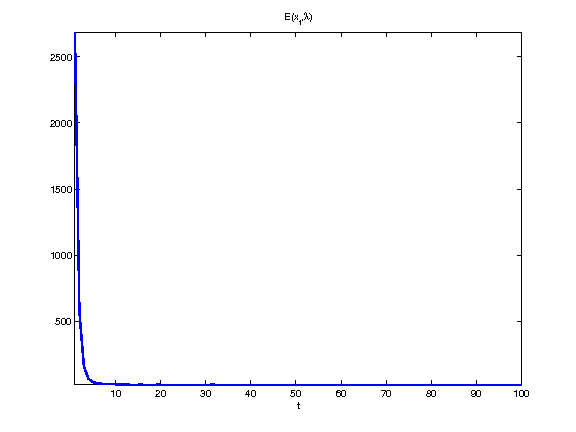In :
%% Insert your code here.


Now that we know how to minimize our functional, we must seek for the most relevent regularization parameter $\la$. Because we know the original image $f_0$, we can compare it to the recovered image $x$ for different values of $\la$. Use the signal-to-noise ratio (SNR) as criterium.

Define a range of acceptable values for $\la$.

In :
lambdaList = logspace( -4, -2, 10 );


Exercise 4

Display the resulting SNR as a function of $\la$. Take the best regularization parameter and display the corresponding recovery.

In :
exo4()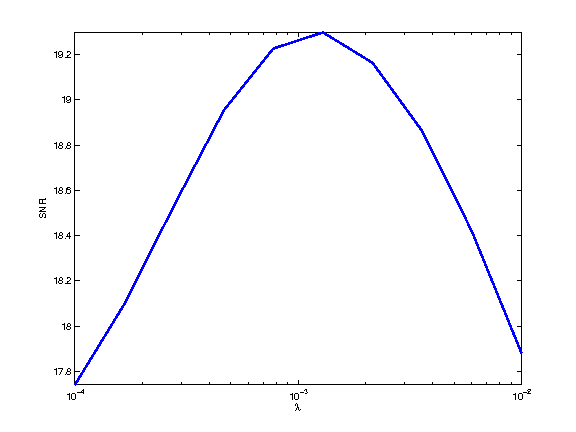In :
%% Insert your code here.


Display the final image, that has been saved in the variable |recov|.

In :
clf
imageplot(recov)
title( sprintf( '\\lambda=%.1e; SNR=%.2fdB', bestLambda, SNRmax ) );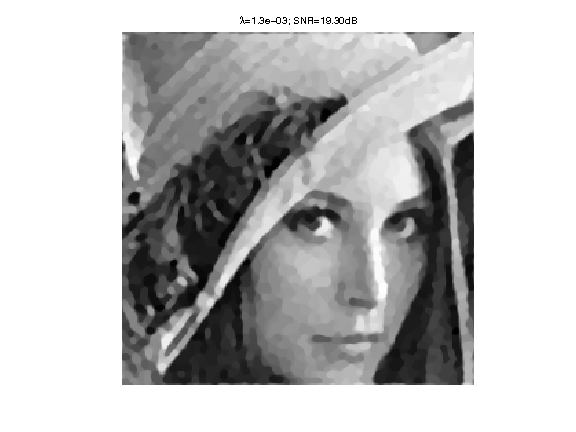## Bonus: Composite Regularization¶

The degradation operator $\Phi$ is very aggressive. To achieve better recovery, it is possible to mix several priors. Let us add a wavelet analysis sparsity prior in the objective $$\umin{x} F(x) + \la \sum_{i=1}^4 G_i(x)  + \mu G_5(x)  \qwhereq G_5(x) = \norm{\Psi x}_1$$ where $\Psi$ is an orthogonal wavelet transform and $\norm{\cdot}_1$ is the $\ell_1$-norm.

The number $n$ of simple functionals is now 5.

In :
n = 5;


Create the wavelet transform $\Psi$.

In :
Jmin = 4;
Psi = @(x)perform_wavortho_transf(x,Jmin,+1);


Define its adjoint $\Psi^*$, where we use the fact that the adjoint of an othogonal operator is its inverse.

In :
Psis = @(x)perform_wavortho_transf(x,Jmin,-1);


Similarly to the $\ell_1-\ell_2$-norm, the proximity operator of the $\ell_1$-norm is a soft-thresholding on each coefficients. Because $\Psi$ is orthogonal, it is also a tight frame operator with bound $b=1$.

Create the proximity operator of $G_5$.

In :
l1norm = @(wx)abs(wx);
proxl1 = @(wx,gamma) max(0,1 - gamma./l1norm(wx) ).*wx;
proxG5 = @(x,gamma) Psis( proxl1(Psi(x),gamma) );


Exercise 5

Solve the composite regularization model. Keep the previous value of $\la$, set $\mu = 10^{-3}$, and perform 500 iterations. Display the results and compare visually to the previous one. Is the SNR significantly improved ? Conclude on the SNR as a quality criterium, and on the usefulness of mixing different regularizations priors.

In :
exo5()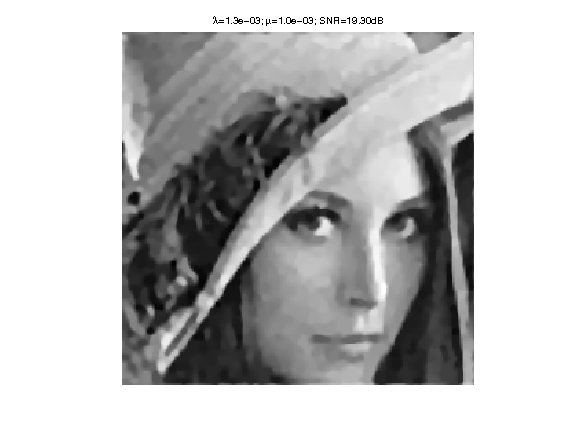In :
%% Insert your code here.